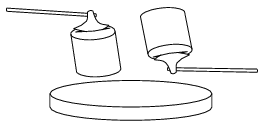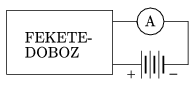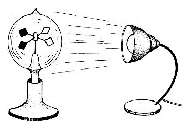Mathematical and Physical Journal
for High Schools
Issued by the MATFUND Foundation
 Already signed up? New to KöMaL?

# KöMaL Problems in Physics, September 2008

Show/hide problems of signs:## Problems with sign 'M'

Deadline expired on October 13, 2008.

M. 289. Investigate how the time of falling of different rectangular shaped sheets of paper depends on the ratio of the sides of the rectangle, if the height from which they are dropped is kept constant. Also keep the area of the rectangles constant and make sure that the plane of each sheet is horizontal when it is dropped.

(6 pont)

statistics## Problems with sign 'P'

Deadline expired on October 13, 2008.

P. 4084. An axe is wedged in a piece of wood. In which case will the wood be split more easily: if the support is hit

X) by the poll (butt) of the axe, or

Y) by the piece of wood?

a) It does not matter by which of the above the support is hit.

b) The support is to be hit by that one which has the smaller mass.

c) The support is to be hit by the piece of wood, because in this case the piece of wood stops, and the axe will penetrate into the wood even more deeply, due to its inertia.

d) In case Y because the axe is made of iron thus its mass is greater.(3 pont)

statistics

P. 4085. A sample of Nitrogen gas is confined into a container at a temperature of 300 K, and at a pressure of 6.105 Pa.

What will the pressure in the container be if 20% of the mass of the Nitrogen is released, and the temperature is increased by 10oC.

(3 pont)

statistics

P. 4086. A black box has two leads. If a zero-centre ammeter and a rechargeable battery of 6 V voltage, whose internal resistance is negligible, are connected to the leads as shown in the figure, the ammeter reads 1 A. If the terminals of the battery are swapped, and connected again into he circuit, then the measured current is 0.5 A.

What is in the box?(4 pont)

statistics

P. 4087. Two satellites of equal mass revolve about the Earth. One of them is at a height of 1000 km and the other is at a height of 2000 km.

Which one has greater kinetic energy and by what factor is it greater than that of the other?

(4 pont)

statistics

P. 4088. The diameter of the rotor blade of a 1-kg model helicopter is 0.4 m. (The rotor blade rotates about a vertical axis.) Estimate the power of the motor which is able to make the helicopter float at a constant height. The density of air is 1.3 kg/m3, the air drag exerted on the body of the helicopter is 10% of the total weight. Consider the speed of the air moved by the rotor blade constant at the whole surface which is swept by the blade.

(5 pont)

statistics

P. 4089. The depth of water in a swimming-pool is 2 m. An incandescent lamp is operated 3 m above the surface of the water. Exactly below the lamp a 1 diopter lens, which has small diameter, is moved up and down. How far is the lens from the surface of the water when the sharp enlarged image of the filament of the lamp appears at the bottom of the pool?

(5 pont)

statistics

P. 4090. A 20-cm long, thin ruler of mass 0.05 kg is lying on the top of a horizontal air-cushioned table. At one moment the ruler is at rest and then at one end of the ruler a horizontal force of magnitude 0.1 N is exerted on it, perpendicularly to the longest side of the ruler. Find the acceleration of the other end of the ruler at which it starts to move.

(5 pont)

statistics

P. 4091. An incandescent lamp emits visible light in the wavelength region of 400 nm and 700 nm. Is it true that the energy of any emitted photon is greater than the average kinetic energy of the atoms of the noble gas with which the bulb is filled? Does the answer depends on with what type of gas the bulb is filled?

(4 pont)

statistics

P. 4092. A laser emits light impulses of wavelength 580 nm and of energy 20 J, which last for 0.5 ms. This light is aimed perpendicularly at a sheet made of caesium, and is focused to a circle of diameter 12 mm. 90% of the light is absorbed and 10% is reflected.

a) How many photons hit the metal sheet during one impulse?

b) Find the pressure of light.

c) Find the maximum of the speed of the electrons which are ejected by the caesium sheet.

(5 pont)

statistics

P. 4093. The light mill (Crookes radiometer) consists of an airtight glass bulb, in which the air pressure is very low, and in which there are four vanes mounted on a vertical spindle, about which they can rotate. The vanes are polished on one side and black on the other. Why and into which direction do the vanes rotate when they are exposed to light?(4 pont)

statistics